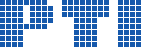Polish Information Processing Society# Maximum Exploratory Equivalence in Trees

## Luka Fürst, Uroš Čibej, Jurij Mihelič

Citation: Proceedings of the 2015 Federated Conference on Computer Science and Information Systems, M. Ganzha, L. Maciaszek, M. Paprzycki (eds). ACSIS, Vol. 5, pages 507518 ()

Full text

Abstract. Many practical problems are modeled with networks and graphs. Their exploration is of significant importance, and several graph-exploration algorithms already exist. In this paper, we focus on a type of vertex equivalence, called exploratory equivalence, which has a great potential to speed up such algorithms. It is an equivalence based on graph automorphisms and can, for example, help us in solving the subgraph isomorphism problem, which is a well-known NP-hard problem. In particular, if a given pattern graph has nontrivial automorphisms, then each of its nontrivial exploratory equivalent classes gives rise to a set of constraints to prune the search space of solutions. In the paper, we define the maximum exploratory equivalence problem. We show that the defined problem is at least as hard the graph isomorphism problem. Additionally, we present a polynomial-time algorithm for solving the problem when the input is restricted to tree graphs. Furthermore, we show that for trees, a maximum exploratory equivalent partition leads to a globally optimal set of subgraph isomorphism constraints, whereas this is not necessarily the case for general graphs.